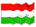These pages are outdated. Me and my webpage have moved to Bristol.

## TeachingSpring Semester, 2013

 Márton Balázs balazs@math.bme.hu

Fall Semester, 2012

 Markov processes and martingales (in Hungarian) Probability Theory for mathematicians, physicists, and other majors (in Hungarian) BSM Probability Theory

Spring Semester, 2012

 Stochastic models (in Hungarian) Probability Theory 2 for mathematicians, physicists, and other majors (in Hungarian) BSM Probability Theory

This was my schedule for the semester.

Fall Semester, 2011

 Markov processes and martingales (in Hungarian) Probability Theory 1 for mathematicians and physicists (in Hungarian) BSM Probability Theory

This was my schedule for the semester.

Spring Semester, 2011

 Stochastic models (in Hungarian) Probability Theory 2. for mathematicians and physicists (in Hungarian) BSM Probability Theory

This was my schedule for the semester.

Fall Semester, 2010

 Markov processes and martingales (in Hungarian) Problem solving in mathematics (in Hungarian) Probability Theory 1. for mathematicians and physicists (in Hungarian) BSM Probability Theory

This was my schedule for the semester.

Spring Semester, 2010

 Mathematics E1 exercise classes (in Hungarian) Mathematics E2 exercise classes (in Hungarian) Mathematics A3 exercise classes (in Hungarian) BSM Probabilty Theory

This was my schedule for the semester.

Fall Semester, 2009

 Problem solving in mathematics (in Hungarian) Probability Theory 1. for mathematicians and physicists (in Hungarian) Probability Theory 1. exercise classes for mathematicians (in Hungarian) Probability Theory 1. exercise classes for physicists (in Hungarian) BSM Probability Theory

Spring Semester, 2009

 Probability Theory 2 (R 8:30-10:00am, J302) (in Hungarian) Mathematics 2. for architect students (W 10:15am-12:00 and F 8:30-10:00am, K220B) (in Hungarian) BSM Probability Theory (M 10:15-11:00am, T 12:15-14:00pm, BSM 105)

Fall Semester, 2008

 Probability Theory 1. for mathematicians and physicists (M: Z F06, 12:15-1:45pm) (in Hungarian) Probability Theory 1. exercise classes for mathematicians (M: H 406, 2:15 - 3:45pm) (in Hungarian) Probability Theory 1. exercise classes for physicists (F: H 601, 10:15am - 11:45) (in Hungarian) BSM Probability Theory (BSM 104, T 10:30-11:15am, W 10:15am-12:00)

Spring Semester, 2008

 BSM Probability Theory (BSM 104, M 10:15-11:45am, F 12:15-1:00pm) Mathematics A2 exercise classes (T: K a52, F: K a52A 8:30-10:00am) (in Hungarian)

Fall Semester, 2007

 Differential Equations for Physicists exercise classes (Z 205, W 12:15am-2:00pm) (in Hungarian) Mathematics A3 in English exercise classes (K 338, M 8:15am-10:00am)

Spring Semester, 2007

 Mathematics E2 exercise classes (W 10:15am-12:00pm) (in Hungarian) Mathematics A2 exercise classes (F 10:15am-12:00pm) (in Hungarian)

Fall semester, 2006

 Mathematics A3 in English lecture (M 10:15am-12:00) and exercise classes (W 3:15pm-5:00pm) Architect Mathematics III. in English exercise classes (T 8:15am-10:00am) Mathematics A4 in Hungarian exercise classes (R 12:15pm-2:00pm) (in Hungarian)[Home] | [Publications] | [Talks] | [Me] | [Utilities] | [Links] | [Teaching]